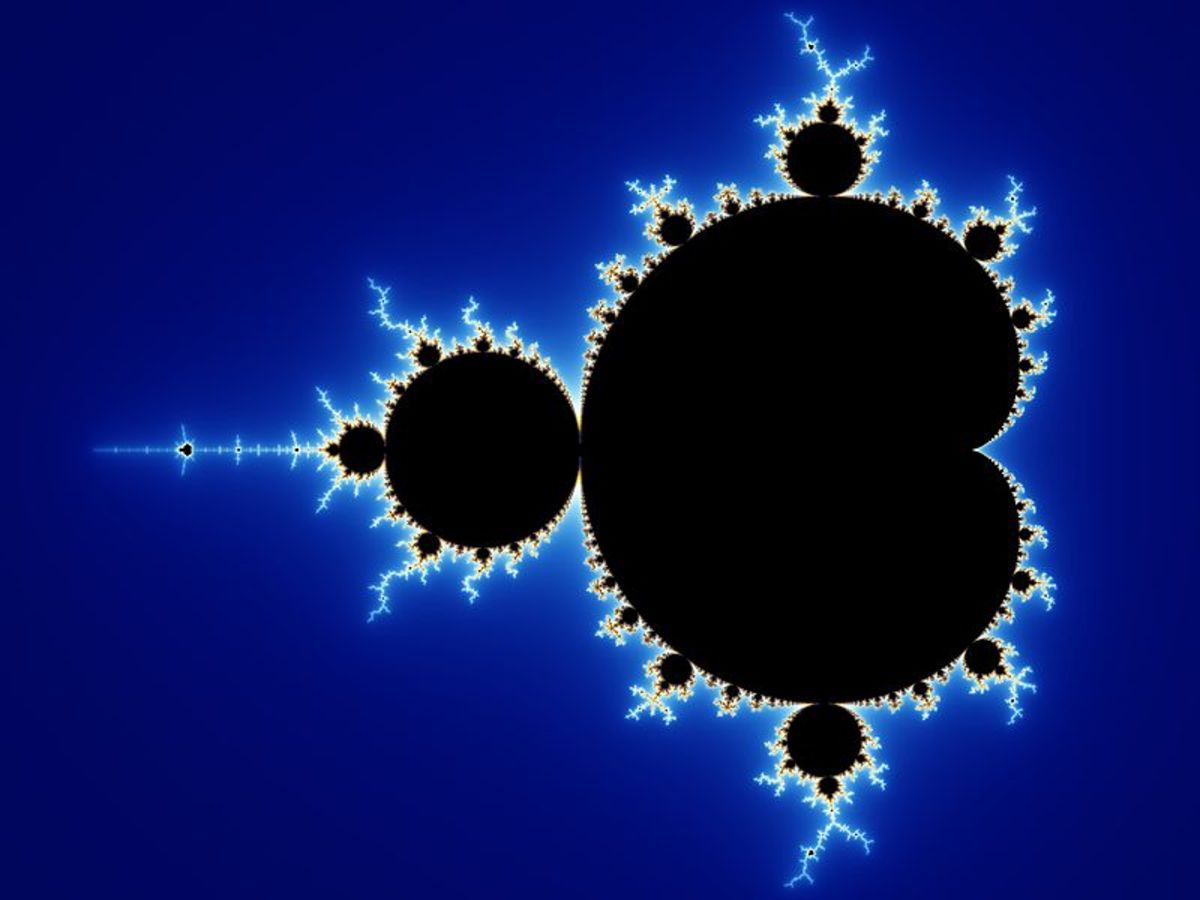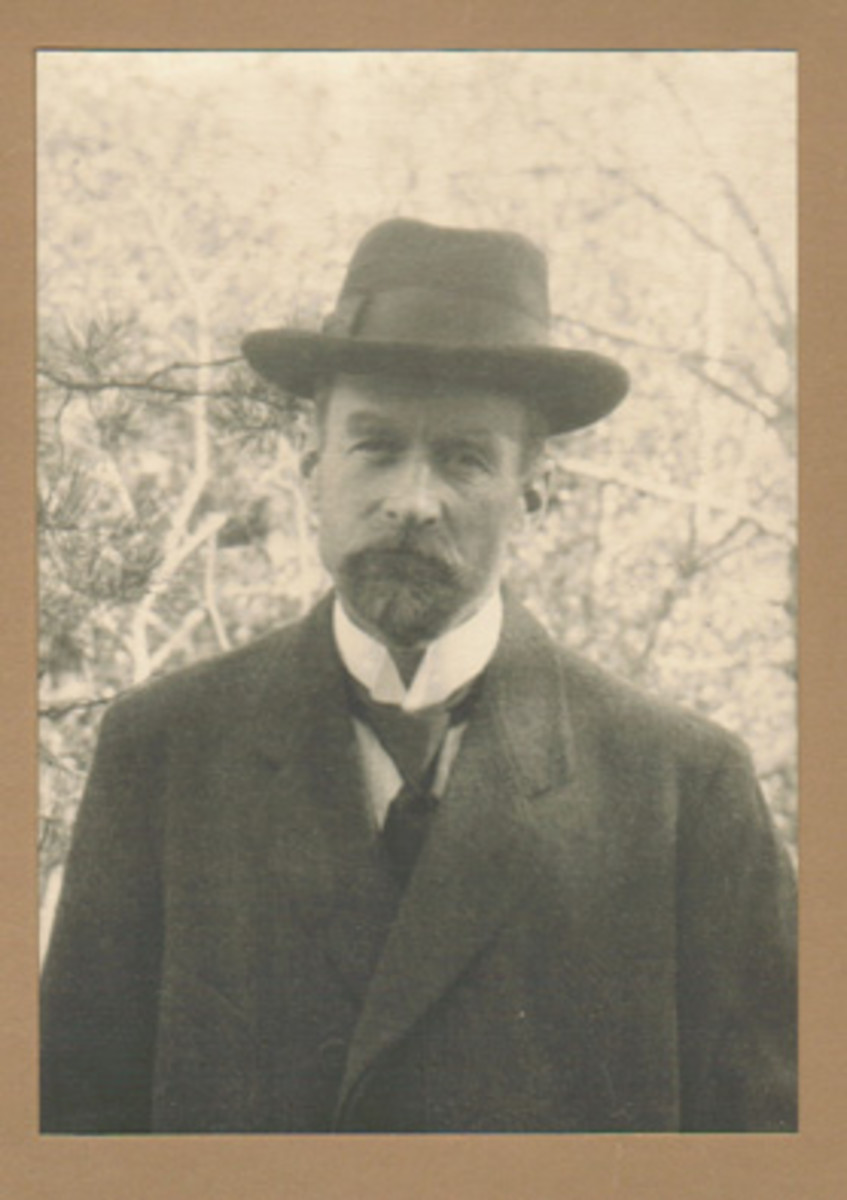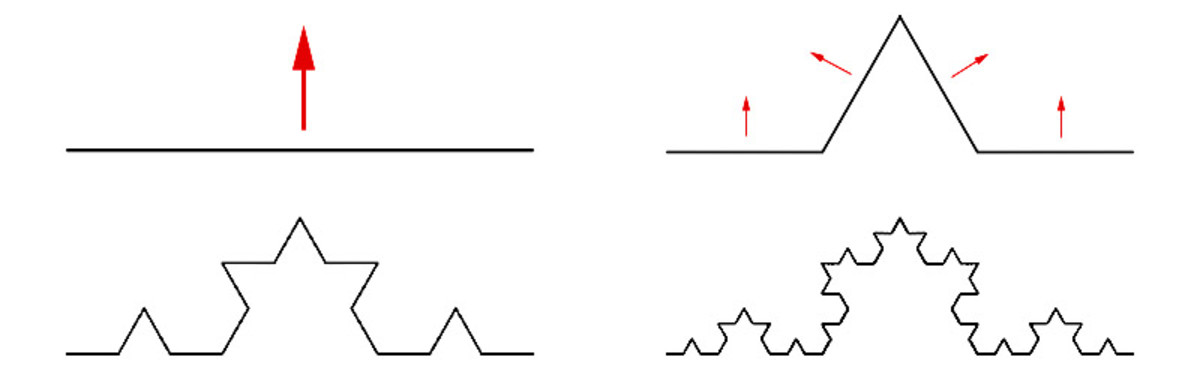# Three Interesting Fractals From Koch, Sierpinski and Cantor

• Author:
• Updated date:

I am a former maths teacher and owner of DoingMaths. I love writing about maths, its applications, and fun mathematical facts.The Mandelbrot SetWolfgang Beyer - https://commons.wikimedia.org/wiki/File:Mandel_zoom_00_mandelbrot_set.jpg

## What Are Fractals?

To formally define fractals would involve delving into some fairly complex mathematics, which is beyond the scope of this article. However, one of the main properties of fractals, and the one most easily recognised in popular culture, is their self-similarity. This self-similarity means that as you zoom in on a fractal you see parts that are similar to other larger parts of the fractal.

Another important part of fractals is their fine structure, i.e. however far you zoom in, there is still detail to be seen.

These properties will both become more evident as we look at some examples of my favourite fractals.

### Three Famous Types of Fractals

1. The Middle Third Cantor Set
2. The Koch Curve
3. The Sierpinski Triangle

## 1. The Middle Third Cantor Set

One of the easiest fractals to construct, the middle third Cantor set, is a fascinating entry-point to fractals. Discovered by the Irish mathematician Henry Smith (1826 - 1883) in 1875, but named for the German mathematician Georg Cantor (1845 - 1918) who first wrote about it in 1883, the middle third Cantor set is defined as such:

• Let E0 be the interval [0,1]. This can be represented physically as a number line from 0 to 1 inclusive and containing all real numbers.
• Delete the middle third of E0 to give the set E1 consisting of the intervals [0,1/3] and [2/3,1].
• Delete the middle third of each of the two intervals in E1 to give E2 consisting of the intervals [0,1/9], [2/9,1/3], [2/3,7/9] and [8/9,1].
• Continue as above, deleting the middle third of each interval as you go.

It can be seen from our examples so far that the set Ek is made up of 2k intervals each of length 3-k.The First Seven Iterations in Creating the Middle Third Cantor Set

The middle third Cantor set is then defined as the set of all numbers in Ek for all integers k. In pictorial terms, the more stages of our line we draw and the more middle thirds we remove, the closer we get to the middle third Cantor set. As this iterative process goes on to infinity, we can never actually draw this set, we can only draw approximations.

### Self-Similarity in the Cantor Set

Earlier in this article, I mentioned the idea of self-similarity. This can be easily seen in our Cantor set diagram. The intervals [0,1/3] and [2/3,1] are exactly the same as the original interval [0,1] but each shrunk to a third of the size. The intervals [0,1/9], [2/9,1/3], etc. are also identical, but this time each is 1/9 of the size of the original.

The middle third Cantor set also starts to illustrate another interesting property of fractals. By the usual definition of length, the Cantor set has no size. Consider that 1/3 of the line is removed in the first step, then 2/9, then 4/27 etc. removing 2n/3n+1 each time. The sum to infinity of 1/3 + 2/9 + 4/27 + ... = 1 and our original set had size 1, so we are left with an interval of size 1 − 1 = 0.

However, by the method of constructing the Cantor set, there must be something left (as we always leave behind the outer thirds of each remaining interval). There is actually an uncountably infinite number of points left. This disparity between the usual definitions of dimensions (topological dimensions) and 'fractal dimensions' is a large part of defining fractals.Helge von Koch (1870 - 1924)
Scroll to Continue

## 2. The Koch Curve

The Koch curve, which first appeared in a paper by Swedish mathematician Helge von Koch, is one of the most recognisable fractals and also very easily defined.

• As before, let E0 be a straight line.
• Set E1 is defined by removing the middle third of E0 and replacing it with the other two sides of an equilateral triangle.
• To construct E2 we do the same again to each of the four edges; remove the middle third and replace with an equilateral triangle.
• Keep repeating this to infinity.

As with the Cantor set, the Koch curve has the same pattern repeating itself on many scales, i.e. no matter how far in you zoom, you still get the exact same detail.The First Four Steps in the Construction of a Koch Curve

### The Von Koch Snowflake

If we fit three Koch curves together we get a Koch snowflake which has another interesting property. In the diagram below, I have added a circle around the snowflake. It can be seen by inspection that the snowflake has a smaller area than the circle as it fits completely inside it. It therefore has a finite area.

However, because each step of the construction of the curve is increasing each side length, each side of the snowflake has infinite length. We therefore have a shape with infinite perimeter but only finite area.

## 3. Sierpinski Triangle (Sierpinski Gasket)

The Sierpinski triangle (named after the Polish mathematician Waclaw Sierpinski (1882 - 1969)) is another easily constructed fractal with self-similar properties.

• Take a filled-in equilateral triangle. This is E0.
• To create E1, split E0 into four identical equilateral triangles and remove the one in the centre.
• Repeat this step for each of the three remaining equilateral triangles. This leaves you with E2.
• Repeat to infinity. To make Ek, remove the middle triangle from each of the triangles of Ek−1.The First Five Steps in the Creation of the Sierpinski Triangle

It can be seen quite easily that the Sierpinski triangle is self-similar. If you zoom in on any individual triangle, it will look exactly the same as the original picture.

### Connection to Pascal's Triangle

Another interesting fact about this fractal is its link to Pascal's triangle. If you take Pascal's triangle and colour in all of the odd numbers, you get a pattern resembling the Sierpinski triangle.

As with the Cantor set, we also get an apparent contradiction with the usual method of measuring dimensions. As each stage of the construction removes a quarter of the area, each stage is 3/4 of the size of the previous one. The product 3/4 × 3/4 × 3/4 × ... tends towards 0 as we go, hence the area of the Sierpinski triangle is 0.

However, each step of the construction is still leaving 3/4 of the previous step behind, hence there must be something left. Again, we have a disparity between the usual measure of dimension and the fractal dimension.